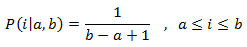# uniform_int_distribution in c++

Unifoirm_int_distribution is a class template. uniform _int_distribution or you can say random number distribution, produces an integer value by using the uniform discrete distribution which is this function:This distribution produces random integers in a range of a and b [a, b]. In other words it returns a new random number that follows the parameters. Since it is a class template, we have to call its member function operator to produce a random value. uniform_int_distribution is used for generating random numbers within a range and the range is in between a and b.

Member types

 Member type Definition Notes result_type The first template parameter (IntType) The type of the numbers generated (defaults to int) param_type The type returned by member param

Member functions

All the member functions given in the table are public member function.

 (constructor) Construct uniform discrete distribution operator() Generate random number reset Reset distribution param Distribution paramters min Minimum value max Maximum value

Distribution parameters

 a Lower range b Upper range

Return value

It returns a random integer value since it is generating a random number.

Examples

``````// uniform_int_distribution::operator()
#include <iostream>
#include <chrono>
#include <random>

int main()
{
// construct a trivial random generator engine from a time-based seed:
unsigned seed = std::chrono::system_clock::now().time_since_epoch().count();
std::default_random_engine generator (seed);

std::uniform_int_distribution<int> distribution(1,10);

std::cout << "some random numbers between 1 and 10: ";
for (int i=0; i<10; ++i)
std::cout << distribution(generator) << " ";

std::cout << std::endl;

return 0;
}``````

Output

`some random numbers between 1 and 10: 2 4 8 9 5 8 1 6 4 10`
` `
`Second example`
``````// uniform_int_distribution
#include <iostream>
#include <random>

int main()
{
const int nrolls = 10000; // number of experiments
const int nstars = 95;    // maximum number of stars to distribute

std::default_random_engine generator;
std::uniform_int_distribution<int> distribution(0,9);

int p={};

for (int i=0; i<nrolls; ++i) {
int number = distribution(generator);
++p[number];
}

std::cout << "uniform_int_distribution (0,9):" << std::endl;
for (int i=0; i<10; ++i)
std::cout << i << ": " << std::string(p[i]*nstars/nrolls,'*') << std::endl;

return 0;
}``````

Output

```uniform_int_distribution (0,9):
0: *********
1: *********
2: *********
3: *********
4: *********
5: *********
6: *********
7: *********
8: *********
9: *********```